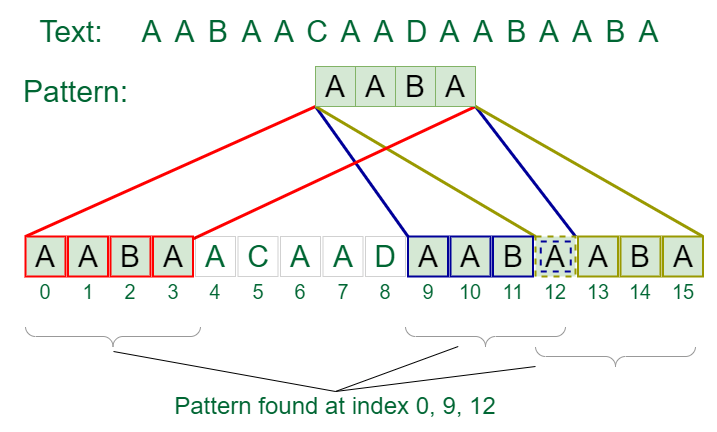Open in App
Not now

# Naive algorithm for Pattern Searching

• Difficulty Level : Easy
• Last Updated : 10 Jan, 2023

Given a text of length N txt[0..N-1] and a pattern of length M pat[0..M-1], write a function search(char pat[], char txt[]) that prints all occurrences of pat[] in txt[]. You may assume that N > M.
Examples:

Input:  txt[] = “THIS IS A TEST TEXT”, pat[] = “TEST”
Output: Pattern found at index 10

Input:  txt[] =  “AABAACAADAABAABA”, pat[] =  “AABA”
Output: Pattern found at index 0, Pattern found at index 9, Pattern found at index 12Pattern searching

Pattern searching is an important problem in computer science. When we do search for a string in a notepad/word file or browser or database, pattern searching algorithms are used to show the search results.

## Naive Pattern Searching algorithm:

Slide the pattern over text one by one and check for a match. If a match is found, then slide by 1 again to check for subsequent matches.

## C

 `// C program for Naive Pattern Searching algorithm``#include ``#include `` ` `void` `search(``char``* pat, ``char``* txt)``{``    ``int` `M = ``strlen``(pat);``    ``int` `N = ``strlen``(txt);`` ` `    ``/* A loop to slide pat[] one by one */``    ``for` `(``int` `i = 0; i <= N - M; i++) {``        ``int` `j;`` ` `        ``/* For current index i, check for pattern match */``        ``for` `(j = 0; j < M; j++)``            ``if` `(txt[i + j] != pat[j])``                ``break``;`` ` `        ``if` `(j``            ``== M) ``// if pat[0...M-1] = txt[i, i+1, ...i+M-1]``            ``printf``(``"Pattern found at index %d \n"``, i);``    ``}``}`` ` `// Driver's code``int` `main()``{``    ``char` `txt[] = ``"AABAACAADAABAAABAA"``;``    ``char` `pat[] = ``"AABA"``;``   ` `      ``// Function call``    ``search(pat, txt);``    ``return` `0;``}`

## C++

 `// C++ program for Naive Pattern``// Searching algorithm``#include ``using` `namespace` `std;`` ` `void` `search(``char``* pat, ``char``* txt)``{``    ``int` `M = ``strlen``(pat);``    ``int` `N = ``strlen``(txt);`` ` `    ``/* A loop to slide pat[] one by one */``    ``for` `(``int` `i = 0; i <= N - M; i++) {``        ``int` `j;`` ` `        ``/* For current index i, check for pattern match */``        ``for` `(j = 0; j < M; j++)``            ``if` `(txt[i + j] != pat[j])``                ``break``;`` ` `        ``if` `(j``            ``== M) ``// if pat[0...M-1] = txt[i, i+1, ...i+M-1]``            ``cout << ``"Pattern found at index "` `<< i << endl;``    ``}``}`` ` `// Driver's Code``int` `main()``{``    ``char` `txt[] = ``"AABAACAADAABAAABAA"``;``    ``char` `pat[] = ``"AABA"``;``   ` `      ``// Function call``    ``search(pat, txt);``    ``return` `0;``}`` ` `// This code is contributed``// by Akanksha Rai`

## Java

 `// Java program for Naive Pattern Searching`` ` `public` `class` `NaiveSearch {`` ` `    ``static` `void` `search(String pat, String txt)``    ``{``        ``int` `l1 = pat.length();``        ``int` `l2 = txt.length();``        ``int` `i = ``0``, j = l2 - ``1``;`` ` `        ``for` `(i = ``0``, j = l2 - ``1``; j < l1;) {`` ` `            ``if` `(txt.equals(pat.substring(i, j + ``1``))) {``                ``System.out.println(``"Pattern found at index "``                                   ``+ i);``            ``}``            ``i++;``            ``j++;``        ``}``    ``}``     ` `      ``// Driver's code``    ``public` `static` `void` `main(String args[])``    ``{``        ``String pat = ``"AABAACAADAABAAABAA"``;``        ``String txt = ``"AABA"``;``     ` `          ``// Function call``        ``search(pat, txt);``    ``}``}``// This code is contributed by D. Vishnu Rahul Varma`

## Python3

 `# Python3 program for Naive Pattern``# Searching algorithm`` ` ` ` `def` `search(pat, txt):``    ``M ``=` `len``(pat)``    ``N ``=` `len``(txt)`` ` `    ``# A loop to slide pat[] one by one */``    ``for` `i ``in` `range``(N ``-` `M ``+` `1``):``        ``j ``=` `0`` ` `        ``# For current index i, check``        ``# for pattern match */``        ``while``(j < M):``            ``if` `(txt[i ``+` `j] !``=` `pat[j]):``                ``break``            ``j ``+``=` `1`` ` `        ``if` `(j ``=``=` `M):``            ``print``(``"Pattern found at index "``, i)`` ` ` ` `# Driver's Code``if` `__name__ ``=``=` `'__main__'``:``    ``txt ``=` `"AABAACAADAABAAABAA"``    ``pat ``=` `"AABA"``     ` `    ``# Function call``    ``search(pat, txt)`` ` `# This code is contributed``# by PrinciRaj1992`

## C#

 `// C# program for Naive Pattern Searching``using` `System;`` ` `class` `GFG {`` ` `    ``public` `static` `void` `search(String txt, String pat)``    ``{``        ``int` `M = pat.Length;``        ``int` `N = txt.Length;`` ` `        ``/* A loop to slide pat one by one */``        ``for` `(``int` `i = 0; i <= N - M; i++) {``            ``int` `j;`` ` `            ``/* For current index i, check for pattern``            ``match */``            ``for` `(j = 0; j < M; j++)``                ``if` `(txt[i + j] != pat[j])``                    ``break``;`` ` `            ``// if pat[0...M-1] = txt[i, i+1, ...i+M-1]``            ``if` `(j == M)``                ``Console.WriteLine(``"Pattern found at index "``                                  ``+ i);``        ``}``    ``}`` ` `    ``// Driver's code``    ``public` `static` `void` `Main()``    ``{``        ``String txt = ``"AABAACAADAABAAABAA"``;``        ``String pat = ``"AABA"``;``       ` `          ``// Function call``        ``search(txt, pat);``    ``}``}``// This code is Contributed by Sam007`

## PHP

 ``

## Javascript

 `// Javascript program for Naive Pattern Searching`` ` `function` `search(txt, pat)``{``    ``let M = pat.length;``    ``let N = txt.length;` `    ``/* A loop to slide pat one by one */``    ``for` `(let i = 0; i <= N - M; i++) {``        ``let j;` `        ``/* For current index i, check for pattern ``        ``match */``        ``for` `(j = 0; j < M; j++)``            ``if` `(txt[i + j] != pat[j])``                ``break``;` `        ``// if pat[0...M-1] = txt[i, i+1, ...i+M-1]``        ``if` `(j == M)``            ``document.write(``"Pattern found at index "` `+ i + ``"
"``);``    ``}``}`` ` `let txt = ``"AABAACAADAABAAABAA"``;``let pat = ``"AABA"``;``search(txt, pat);`

Output

```Pattern found at index 0
Pattern found at index 9
Pattern found at index 13 ```

Time Complexity: O(N2)
Auxiliary Space: O(1)

## What is the best case of Naive algorithm for Pattern Searching?

The best case occurs when the first character of the pattern is not present in the text at all.

## C

 `txt[] = ``"AABCCAADDEE"``;``pat[] = ``"FAA"``;`

The number of comparisons in the best case is O(N).

## What is the worst caseof Naive algorithm for Pattern Searching?

The worst case of Naive Pattern Searching occurs in the following scenarios.
1) When all characters of the text and pattern are the same.

## C

 `txt[] = ``"AAAAAAAAAAAAAAAAAA"``;``pat[] = ``"AAAAA"``;`

2) Worst case also occurs when only the last character is different.

## C

 `txt[] = ``"AAAAAAAAAAAAAAAAAB"``;``pat[] = ``"AAAAB"``;`

The number of comparisons in the worst case is O(M * (N – M + 1)). Although strings which have repeated characters are not likely to appear in English text, they may well occur in other applications (for example, in binary texts). The KMP matching algorithm improves the worst case to O(N). We will be covering KMP in the next post. Also, we will be writing more posts to cover all pattern searching algorithms and data structures.